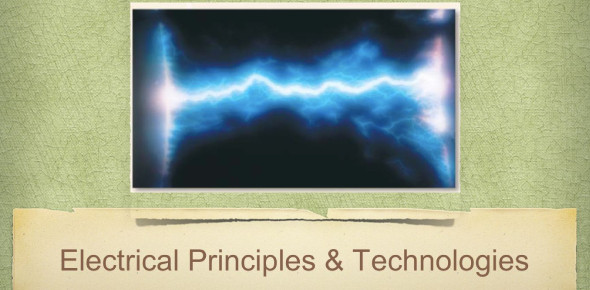# A Trivia Quiz On Electrical Principles And Technologies!

32 Questions | Attempts: 974
ShareSettingsIntererested to learn about electrical principles and technologies? This quiz can spark your interest. With this quiz, you will have to learn which conductors have no resistance to electron flow, what happens to an abundance of charged particles in a solution, an example of a static electrical charge, and how you can help to diminish static cling. If you are searching for information on electrical principles and technologies, look no further than this quiz.

• 1.
These types of conductors have no resistance to electron flow, which is why they are known to be perfect conductors. They are
• A.

Superconductors

• B.

Metallic conductors

• C.

Magnetic conductors

• D.

Resistance-less conductor

• 2.
The more charged particles in a solution ______________.
• A.

The more voltage it produces

• B.

The more resistance there is

• C.

The less resistance there is

• D.

The fewer molecules it has

• 3.
__________ is the direction of a magnetic or electric field.
• 4.
The ___________ (one or two words) in an electric motor attracts the armature to create motion; in which it creates electricity.
• 5.
A generator stores electric current.
• A.

True

• B.

False

• 6.
The movement of charges from an object to another without further movement is called _____________.
• 7.
An example of a static electrical charge is _______.
• A.

Lightning

• B.

Batteries

• C.

Current

• D.

Ohms

• 8.
Why do objects attract to one another?
• A.

Opposite charges

• B.

Similar charges

• C.

Electrons

• D.

Neutrons

• 9.
How does spraying water on laundry help reduce static cling?
• A.

The water absorbs electrical charge

• B.

The water contains protons

• C.

Water is a magnet

• D.

The charges leave onto a different object with opposite charges

• 10.
When electrons discharge, they go into the ground via a _________ wire.
• 11.
A ________ is designed to interrupt the power to a circuit when the current flow exceeds safe levels.
• 12.
A _________ occurs when there is a low resistance connection between two conductors supplying electrical power to any circuit.
• A.

Short circuit

• B.

Malfunction

• C.

Electrical shock

• D.

Spark

• 13.
____________ is the energy carried by charged particles.
• 14.
How much current is created when a 30 V battery creates a current through a 15 Ω resistor?
• 15.
The measurement used to measure voltage is ________.
• 16.
The measurement used to measure current is _______.
• 17.
The process of which you use a small battery to split molecules into their elements is called _________.
• A.

Electrolysis

• B.

Hydrolysis

• C.

Energy conversion

• D.

Energy transfer

• 18.
A _________, is a paste or liquid that conducts electricity because it contains chemicals that form ions.
• A.

Electrolyte

• B.

Battery

• C.

Dry cell

• D.

Wet cell

• 19.
Who had made the first practical battery by piling copper and zinc disks on top of each other, and then seperating them with electrolyte-soaked paper discs.
• A.

Alessandro Volta

• B.

• C.

Van de Graaff

• D.

Ohm

• 20.
A dry cell is different from a wet cell because it is ____________.
• A.

Rechargeable

• B.

Nonchargeable

• C.

Dry

• D.

Wet

• 21.
The longer the wire is, the ______ its resistance.
• A.

Lower

• B.

Greater

• C.

Wider

• D.

Thinner

• 22.
The thicker the wire is, the ________ its resistance.
• A.

Lower

• B.

Greater

• 23.
The ______  a wire is, the lower the resistance.
• A.

Cooler

• B.

Hotter

• C.

More conductive

• 24.
A _________ is a continuous resistor used to regulate electric current, it is also used to change the current flow.
• A.

Rheostat

• B.

Voltmeter

• C.

Ammeter

• D.

Battery

• 25.
What is the total resistance of a parallel circuit with two 20-ohm resistors and a 10-ohm resistor?

## Related TopicsBack to top
×

Wait!
Here's an interesting quiz for you.